# NP and the difficulty of calculation

For an algorithm, it is generally necessary to calculate its time complexity and space complexity to measure its efficiency. However, there are some extremely difficult problems, and it is difficult to prove that they cannot be solved by effective algorithms. Therefore, a reduction method is proposed to prove that problem X is at least as difficult as problem Y.
Reference books: “Algorithm Design” and “Algorithm Design”

## 1 Polynomial time reduction

### 1.1 The concept of reduction

When studying the difficulty of different problems, I hope to express “problem X is at least as difficult as problem Y”. This is reduction.

### 1.2 Polynomial time reduction

In the calculation model, it is assumed that problem X can be solved in polynomial time. If there is a “black box” to solve problem X, assuming that the black box that solves problem X can be solved by polynomial order, problem Y can be solved, then problem X has enough ability to solve problem Y, which is called “Y polynomial time can be reduced To X”.
Recorded as: Y \leq_p X, pronounced “Y polynomial time can be reduced to X” or “X is at least as difficult as Y.”
Then, we can get the following theorem:
Theorem 1. Assuming Y \leq_p X, if it can be solved in polynomial time, it can also be solved in polynomial time.
Theorem 2. Suppose Y \leq_p X, if it cannot be solved in polynomial time, then X cannot be solved in polynomial time.

### 1.3 Reduction between independent sets and vertex covers

【Independent set problem (Independent set)】
An independent set (also called a stable set) is a set formed by some pair of non-adjacent vertices in a graph. That is, there is no edge between any two points in the vertex set. As shown in Figure 1, the red point sets are independent sets, and any two red vertices have no edges.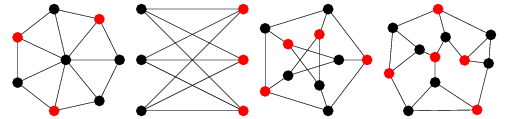Figure 1 Independent set (red)
【Vertex Cover Problem】
The vertex cover of the graph is also a collection of points. Each edge in the graph has at least one vertex in the point set. As shown in Figure 2, the black point sets are all vertex cover sets, and each edge of the graph has at least one vertex in the point set.Figure 2 Vertex cover set (black)
【Reduction between independent set and vertex cover】
Independent set problem: Given a graph G and a number k, does G contain an independent set with a size of at least k?
Vertex cover problem: Given a graph G and a number k, does G contain a vertex cover of size k?
Prove that the difficulty of the two problems are equivalent.
Theorem 3: Let G=(V,E) be a graph, S\subseteq V, then S is an independent set if and only if its complement V-S is a vertex cover.
prove:
Suppose S is an independent set, consider any edge, because S is an independent set, u and v cannot both be in S, so one of u and v must be in V-S. Then at least one end point of each article is in V-S, that is, V-S is a point covering set.
In turn, suppose that V-S is a vertex cover. Consider any two vertices u and v in S. If there is an edge e between them, the two endpoints of e are not in V-S, which contradicts the assumption that V-S is a vertex cover. Therefore, if there is no edge between any two vertices in S, then S is an independent set.
[Independent set \leq_p vertex cover]
Proof: If there is a black box that solves the vertex cover, by asking whether the black box G has a vertex cover of at most n-k, it can be determined whether G has an independent set of at least k.
[Vertex Cover\leq_pIndependent Set]
Proof: If there is a black box that solves the independent set, then by asking whether the black box G has an independent set of at most n-k, it can be determined whether G has an independent set of at least k.

### 1.4 Vertex Cover to Set Cover Reduction

The vertex covering problem can be regarded as a “covering problem”, and the goal is to “cover” all the edges in the graph with as few vertices as possible.
[Set Coverage Problem]
The set coverage problem can be described as:
Given a set of n elements U, subsets of U S_1,…,S_m and the number k, there is a set of subsets in these subsets, whose union is equal to the entire U and contains at most k subsets?
Figure 3 shows an example of a set covering problem.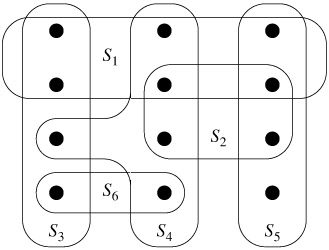Figure 3 Examples of set covering problems
In Figure 3, there are 3 subsets (that is, the number k is 3) {S_3,S_4,S_5}, which is not equal to the entire U.
[Vertex Cover\leq_pSet Cover]
The vertex cover problem can be reduced to the set cover problem.
That is: if there is a black box covered by the solution set, the vertex cover problem can be solved by inputting an instance of the vertex cover and calling this black box.
This construction method can be used: for each vertex i \in V, set S_i \subseteq U to be all edges in G connected to vertex i. According to this method, a set is constructed for each vertex.
Then U can be covered by at most k sets in S_1,S_2,S_3,…,S_n if and only if G has vertices with a size of at most k.
Because if S_{i_1},S_{i_2},S_{i_3},…,S_{i_l} are sets of l \leq k covering U, then each item in G All edges can be associated with one of the vertices i_1,i_2,i_3,…,i_l, so {i_1,i_2,i_3,…,i_l} is the size of G as l \leq The vertex cover of k.
Therefore, given an instance of vertex cover, use the above construction method to construct an instance of set cover, and input it into the black box, and answer yes if and only if the black box answers yes.

### 1.5 Independent set to set packaging reduction

The independent set problem can be regarded as a “packing problem”, and its purpose is to pack more vertices.
【Collection packaging problem】
The collection packaging problem can be described as:
Given a set of n elements U, a subset of U S_1,S_2,…,S_m and a number k, ask that there are at least k in these subsets. not intersect?
[Independent collection \leq_p collection packaging]
Set cover is a natural promotion of vertex cover, and set packaging is a natural promotion of independent sets. Using the same construction method and similar proof method, it can be proved that the independent set problem can be reduced to the set packing problem.

## 2 Satisfaction problem

### 2.1 SAT and 3-SAT

【SAT Questions】
Call the Boolean satisfiability problem SAT:
Given a set of clauses C_1,C_2,…,C_n on the set of variables X={{x_1,x_2,…,x_n}}, is there a satisfactory truth value assignment?
For example, there are 3 clauses: (x_1 \vee \overline {x_2} ),(\overline {x_1} \vee \overline {x_3} ),(x_2 \vee \overline {x_3} ), then if you let If all variables are 1, then all clauses cannot be satisfied, because the value of the second clause is 0.
【3-SAT Question】
The 3-SAT problem is also called the ternary satisfiability problem. Because each clause contains exactly three items.
The 3-SAT problem can be described as:
Given the set of variables X={{x_1,x_2,…,x_n}} on a set of clauses C_1,C_2,…,C_n, the length of each clause is 3, Is there a true value assignment that satisfies?

### 2.2 3-SAT reduction to independent set problem

[3-SAT \leq_p independent set]
To prove that the 3-SAT problem can be reduced to the independent set, it is necessary to prove that there is a black box about the independent set. By solving the 3-SAT instance, the 3-SAT problem can be solved.
Figure 4 shows an example of reduction from 3-SAT to independent set.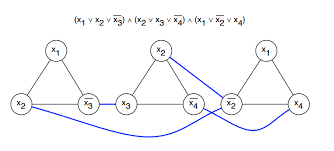Figure 4 Reduction from 3-SAT to independent set
For a clause, as long as the value of one item is true, the value of the entire clause is true.
Then, according to the clauses, the graph can be constructed like this: == For each clause, create three points and connect the three points into a triangle (as shown in the figure above). If there are two clauses with x_1 and \overline x_1, add an edge == between these two nodes (called conflict change, that is, the two sides cannot be selected at the same time).
Then, there is a truth assignment, if and only if one node is taken in each clause, and there is an independent set of size m (the number of clauses).
For example, in Figure 4, there is an independent set {x_1, x_3, x_4} of size 3, and these three values ​​are set to 1, respectively, so that the truth value of all clauses is 1, that is, the The conjunction is 1, this set of clauses is satisfying.

### 2.3 Transitivity of reduction

There is transitivity between reductions.
Theorem 4. If X \leq_p Y, Y \leq_p Z,, then X \leq_p Z.
Through the above reduction, you can get:
3-SAT \leq_p independent set \leq_p vertex cover \leq_p set cover

## 3 P, NP problem

### 3.1 P (polynomial time) problem

For a computational problem, encode its input into a finite binary string s. The decision question accepts the input string s and returns “yes” or “no”. This return value is recorded as A(s). If there is a polynomial function p, so that for each input string s, the calculation of algorithm A on s ends at O(p(|s|)) at most, then A has a polynomial running time. ==P problem is a problem that can be solved in polynomial time ==. P refers to Polynomia.

### 3.2 NP (nondeterministic polynomial time) problem

In order to verify one solution, you need to enter the string s and another “certificate” string t. If the following two properties are true, then B is an effective verification procedure for question X:
(1) B is a polynomial time algorithm with two input variables s and t.
(2) There is a polynomial p such that for each input string s, A(s)=yes if and only if there is a string t such that |t|≦p(|s|) and B(s,t)=yes.
==NP question is a question that can verify the correctness of the answer in polynomial time, that is, the set of all questions that have valid verification procedures ==.

### 3.3 The relationship between P problem and NP problem

Theorem 5.P \subseteq NP.
That is, all P problems are NP problems. When a problem is a P problem, we can find the solution of the problem in polynomial time. To verify whether a solution (denoted as t1) is correct, you only need to use polynomial time to solve the problem (denoted as t2), and then compare t1 and t2 to verify whether the answer is correct. That is, polynomial time can be used to verify whether the answer is correct or not. Therefore, the P problem is also an NP problem. It can be seen that the ternary satisfiability problem (3-SAT), independent set problem, and set cover problem are all NP problems.
【Discussion: P=NP?】
No one has used an effective method to prove this problem. At present, the computer community generally believes that P≠NP. So the P problem is a proper subset of the NP problem.

## 4 NPC problem (NP complete problem)

In the absence of progress on the question of whether P=NP, people turn to a relatively, more easily handled problem: which are the most difficult problems in NP?

### 4.1 NPC (NP-Complete) problem

If a problem X is an NP problem, and for all Y \in NP,Y \leq_p X. That is, every problem in NP can be reduced to X, then problem X is said to be NP complete.
✿So, it can be obtained that if there is a problem in NP that is not solvable in polynomial time, then all NP complete problems are not solvable in polynomial time.
And, if Y is a NP complete problem, X belongs to NP and Y \leq_p X, then X is NP complete.

### 4.2 The problem of circuit satisfiability is an NPC problem

【Circuit Satisfaction Problem】
Circuit satisfiability problem is described as: Given a circuit, it is necessary to determine whether there is an assignment to the input so that the output value is 1. If there is such an assignment, the circuit is said to be satisfactory. This assignment is also called a satisfying assignment. Figure 5 shows an example of the circuit satisfiability problem.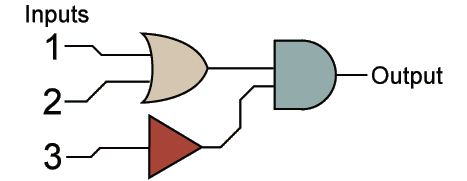Figure 5 Circuit satisfiability problem
On the left side of the figure, from top to bottom are OR gate and NOT gate. The right side of the figure is the AND gate. When 1 and 2 inputs are both 1, and 3 inputs are 0, the output is 1. That is, this circuit is satisfactory.
Theorem 6. The problem of circuit satisfiability is an NPC problem.
The problem of circuit satisfiability is NPC. This theorem was put forward by Cook and Levin in 1971.
It is NPC to prove that the problem of circuit satisfiability is NPC. According to the definition of NPC, the following two points need to be proved:
(1) The problem of circuit satisfiability is an NP problem.
(2) All NP problems can be reduced to circuit satisfiability problems.
prove:
(1) For each input of the circuit, only need to verify whether the result is 1. Therefore, it is possible to verify whether the answer is correct in polynomial time. That is, the problem of circuit satisfiability is an NP problem.
(2) For a problem, we can construct a circuit with input length (|s|+|t|) from the input instance s and certificate t. That is, there are (|s|+|t|) source nodes (input nodes). It is easy to see that there must be a way to design the circuit so that the output of the circuit is 1. That is, the circuit is satisfactory. Shows that all NP problems can be reduced to circuit satisfiability problems.
Therefore, the problem of circuit satisfiability is an NPC problem.

### 4.3 The ternary satisfiability problem is an NPC problem

Theorem 7. If Y is a NP complete problem, X belongs to NP, and Y \leq_p X, then X is NP complete.
It has been proved that 3-SAT is an NP problem, and it can be proved to be NP-complete by proving the satisfiability of the circuit \leq_P 3-SAT.
[Circuit Satisfaction \leq_P 3-SAT]
For a circuit K, for each node v of the circuit, a variable x_v is associated, and x_v represents the true value at that node. There are three types of gate calculations for circuit K: AND, OR, and NOT.
If a node v is a negation gate, its only entry edge comes from node u, then x_v = \overline x_u, which can be done by adding two clauses (x_v \vee x_u) or (\overline x_v \vee \overline x_u) to ensure this.
If a node v is an OR gate, then its 2 edges come from nodes u and w respectively, then there must be x_v = x_u \vee x_w, you can add three clauses (x_v \vee \ overline x_u), (x_v \vee \overline x_w) or (\overline x_v \vee x_u \vee x_w) to ensure this.
If a node v is an AND gate, then its 2 edges come from nodes u and w respectively, then there must be x_v = x_u \wedge x_w, you can add three clauses (\overline x_v \ vee x_u), (\overline x_v \vee x_w) or (x_v \vee \overline x_u \vee \overline x_w) to ensure this.
For the source node, to ensure that it is equal to the specified value, a clause containing only one item x_v or \overline x_v can be added to each source node v to force it to take the specified value. For the output node, add a univariate clause and let it take 1. The structure is thus completed.
It can be proved that the constructed SAT instance is equivalent to the given circuit satisfiability instance.
Since we want to prove the satisfiability of the circuit \leq_P 3-SAT, we also need to convert the clause to an instance with exactly 3 variables.
For clauses with only one item t, the clause can be replaced with (t \vee z_1 \vee z_2);
For a clause with 2 items (t \vee t’), it can be replaced with (t \vee t’\vee z_1).
Obviously, in order to ensure that the newly constructed clause is equivalent to the original clause, it is necessary to ensure that z_1=z_2=0.
To ensure that z_1=z_2=0, for i=1 and 2, you can add clauses (\overline z_i \vee z_3 \vee z_4), (\overline z_i \vee \overline z_3 \vee z_4) , (\overline z_i \vee z_3 \vee \overline z_4), (\overline z_i \vee \overline z_3 \vee \overline z_4), guarantee z_1=z_2=0
Thus completed the construction of the circuit K to 3-SAT. Therefore, the circuit satisfiability is \leq_P 3-SAT. The certificate is complete.
Therefore, according to the 3-SAT \leq_p independent set \leq_p vertex cover \leq_p set cover, it can be obtained that independent set, vertex cover, set packing, and set cover are all NPC problems.

### 4.4 General strategies for proving NPC problems

Given a basic problem X, the basic strategy to prove that it is an NPC is:
(1) Prove that X is an NP problem.
(2) Choose a known NPC problem Y.
(3) Prove Y \leq_p X.

## 5 Sorting problem

Mainly introduce the two sorting problems of Hamilton circle and itinerant salesperson problem.

### 5.1 Itinerant salesperson problem

【Roving Salesperson Question】
There is a traveling salesperson who must visit n cities, which are denoted as v_1,v_2,v_3,…,v_n. The salesperson starts from the city v_1 where he lives, looking for a travel route and visiting all other cities The order in which the city finally returned home. The goal is to keep the distance of the entire travel path as small as possible.
For each pair of cities (v_i,v_j), the distance between the cities is d(v_i,v_j). Moreover, the distance is not symmetric, that is, d(v_i,v_j) \neq d(v_j,v_i). It is also not required that the distances satisfy the triangle inequality. Therefore, the goal of the itinerant salesperson problem is to make the total distance \sum_{j=1}^{n-1}d(v_{i_j},v_{i_{j+1}})+d(v_{i_n},v_ {i_1}) minimum.
The decision form of the itinerant salesperson problem is: Given the set of distances between n cities and the limit D, is there a route that does not exceed D in length?

### 5.2 Hamiltonian circle problem

【Hamilton Circle Problem】
For a directed graph G=(V,E), if the cycle C in G passes through each vertex exactly once, then the cycle C is called a Hamiltonian cycle. That is, the Hamiltonian circle constitutes a “route” that passes through all the vertices without repeating. Figure 6 is a graph with Hamiltonian cycles.Figure 6 A directed graph with Hamiltonian cycles

### 5.3 The Hamiltonian cycle problem is NP complete

Prove that the Hamiltonian circle problem is NPC, which can be obtained by proving 3-SAT\leq_pHamiltonian circle.
【3-SAT\leq_pHamilton Circle】
The construction method is as follows:
(1) For each variable x_i, create 3m+3 vertices. Name it v_{i,1},…,v_{i,3m+3}, and add edges to adjacent vertices (v_{i,j},v_{i,j+1}) and (v_{i,j+1},v_{i,j}). As shown in Figure 7, the red dots and green edges. If x_i is 1, the path P_i is from left to right. Conversely, if x_i is 0, the path P_i is from right to left.
(2) For each variable, add an edge (v_{i,1},v_{i+1,1}),(v_{i,1},v_{i+1,3m+3}),(v_{i,3m+3},v_{i+1,1}),(v_{i,3m+3},v_{i+1,3m+3}).
The structured diagram is shown in Figure 7.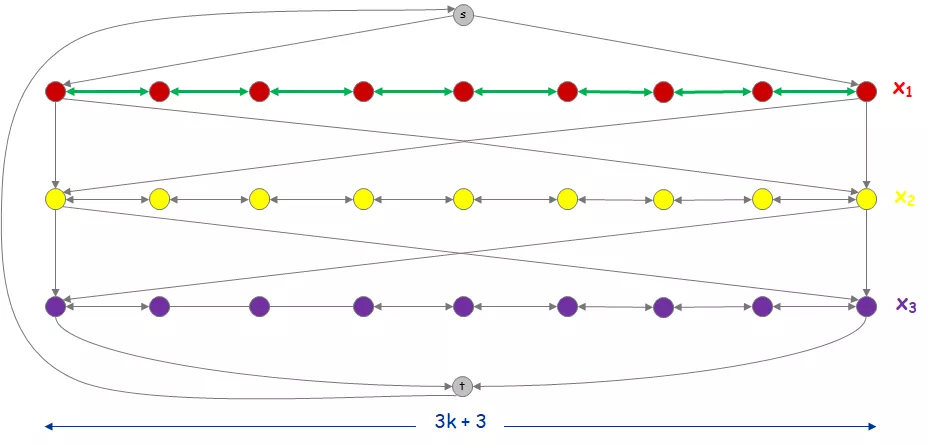Figure 7 Reduction of 3-SAT to Hamiltonian circle, part 1
In Figure 7, we can see that there is a Hamiltonian circuit: starting from t to s, s passes through P_{i}, and finally reaches t, denoted as A.
The clause is then constrained.
(5) For each clause C_a, assuming the clause is C_1=x_1 \vee \overline x_2 \vee x_3, then this clause indicates that the Hamiltonian cycle first passes through P_1 from left to right, and then from right Pass P_2 left, and finally pass P_3 from left to right. As shown in Figure 8, add points and edges.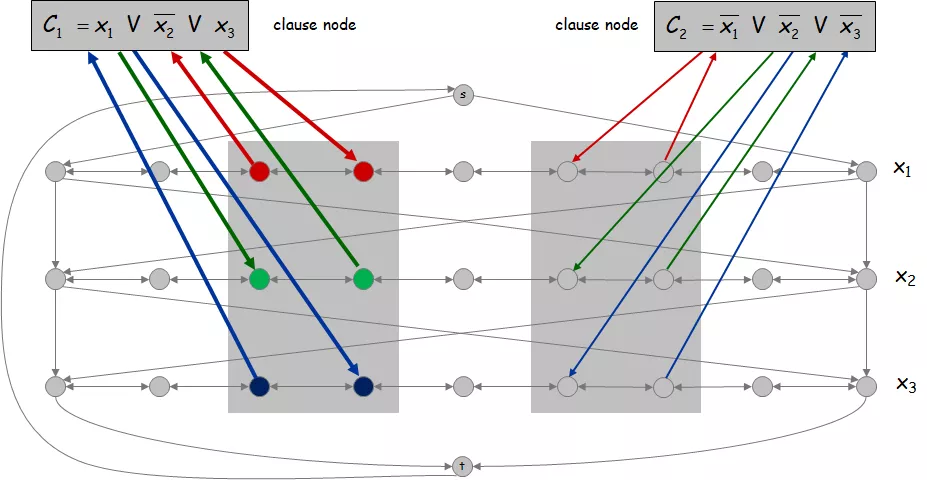Figure 8 Reduction from 3-SAT to Hamiltonian circle, part 2
It can be seen that there are Hamiltonian cycles in the figure if and only if the 3-SAT instance has a satisfactory assignment.
Proof: Assuming that the 3-SAT instance has a satisfactory assignment, in the Hamilton circle given, if x_i is 1 in the satisfactory assignment, then pass P_{i} from left to right, otherwise from right to Pass P_{i} left. For each clause, because it is satisfied, at least one path is passed in the “correct” direction related to the item. Thus the added clause vertex can be passed in the Hamiltonian cycle. Conversely, assuming that there is a Hamiltonian cycle in graph G, all added clause vertices (points added in Figure 8) will be passed. That is, all clauses are satisfied.
Therefore, it can be obtained that the 3-SAT instance is satisfiable if and only if G has a Hamiltonian cycle. Prove the 3-SAT\leq_p Hamiltonian cycle. So the Hamilton circle problem is NPC.

### 5.4 The traveling salesperson problem is NP-complete

First of all, it is easy to prove that the traveling salesperson problem is an NP problem, and its verification program can verify whether the length of the corresponding route exceeds a given limit in polynomial time.
It is possible to prove that the itinerant salesperson problem is NP-complete by proving the Hamilton circle \leq_p itinerant salesperson.
【Hamilton Circle\leq_ptouring salesperson】
For a directed graph G=(V,E), the following example of itinerant salesperson problem is specified: For each point v_i in the graph, there is a city v_{i}’. If there is an edge in G, then d(v_i’,v_j’)=1, otherwise d(v_i’,v_j’)=2.
Then, there is a Hamiltonian circle in G if and only if there is a patrol circuit whose length does not exceed n in this itinerant salesperson problem.
Therefore, in Hamilton circle \leq_p itinerant salesperson, the problem of itinerant salesperson is NPC.

### 5.5 Hamiltonian path problem

【Hamiltonian path problem】
The Hamiltonian path problem is a variant of the Hamiltonian cycle problem. If the path P in the directed graph G contains each vertex exactly once, it is called a Hamiltonian path.

### 5.6 The Hamiltonian path problem is NP-complete

It is proved that the Hamiltonian path is NP-complete and can be reduced to the Hamiltonian path by 3-SAT. This is very similar to the 3-SAT reduction to Hamilton problem (but there is no edge from t to s).

## 6 Division problem

Discuss two division problems, one is the three-dimensional matching problem, and the other is the graph coloring problem. For the three-dimensional matching problem, a search is required to divide the object set into subsets. For the problem of graph coloring, it is required to divide the nodes in the graph.

### 6.1 Three-dimensional matching problem

【Three-dimensional matching problem】
Given three disjoint sets X, Y, Z, the size of all three sets is n. Given a set of triples T \subseteq X \times Y \times Z, the size of the set T is m.
Question: Is there a subset T’of size n in T that happens to contain each element of X, Y, and Z once?
The three-dimensional matching problem is actually a special case of the set covering and set packing problem.

### 6.2 The three-dimensional matching problem is NP-complete

First, it is easy to prove that the three-dimensional matching problem is an NP problem. It only needs to determine whether the size of the set T’is n, and it contains each element of X, Y, and Z once. Prove that the three-dimensional matching problem is NPC, which can be proved by 3-SAT\leq_p three-dimensional matching.
[3-SAT\leq_p3D matching certificate]
Consider an arbitrary 3-SAT instance, there are n variables x_1,x_2,…,x_n and k clauses C_1,C_2,…,C_n. Construct 3DM instance X, Y, Z, T.
For each variable, there is a corresponding part. As shown in Figure 9. Each part has Core (core) element, Tip (edge) element, Triple tuple. The size of each part is 2k. k is the size of the clause.
Then, for each variable x_i, create:
A_i={a_{i,1},a_{i,2},…,a_{i,2k}}.
B_i={b_{i,1},b_{i,2},…,b_{i,2k}}.
t_{i,j}={(a_{i,j},a_{i,j+1},b_{i,j})}.
The structured parts are shown in Figure 9.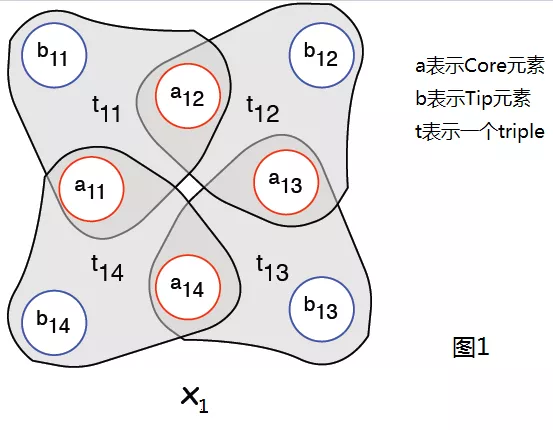Figure 9 Three-dimensional matching parts
For each clause C_t add two elements P_t,P_t’, if C_{t} contains x_{j}, then join the triplet P=(P_t,P_t’,b_{ j,2t}), obviously a C_i has three triples.
If \overline x_j is included, add P=(P_t,P_t’,b_{j, 2t-1}); so the tip tuples contained in the triples of each clause do not conflict. That is, the triples of C_i and C_j do not have the same b element. As shown in Figure 10.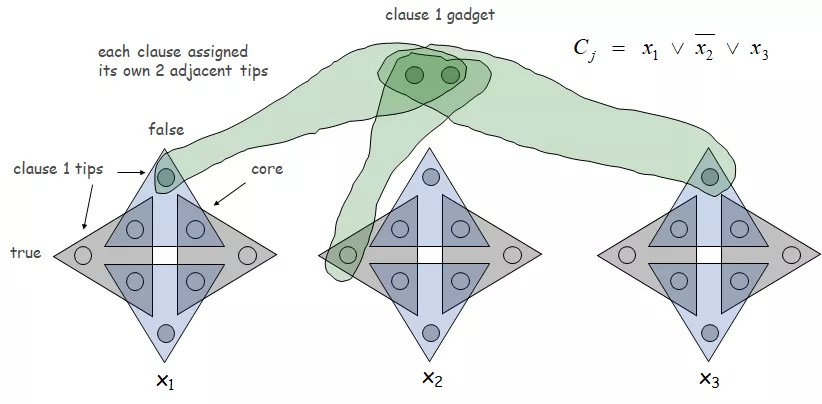Figure 10 Reduction from 3-SAT to three-dimensional matching, part one
Definition, if j in t_{ij} is an even number, then t_{ij} is called an even triplet, and if j is an odd number, t_{ij} is an odd triplet.
If j in b_{i,j} is an even number, then b_{i,j} is called an even tip, and if j is an odd number, then b_{i,j} is called an odd tip.
As you can see, if you want core elements to be included without being included repeatedly, you must either select all even triples or all odd triples. Define that if all even triples are selected, x_{i}=0, otherwise, x_i=1.
Therefore, it can be seen that if a clause is 1, there must be a value of 1, that is, to select all odd triples or all even triples of the variable. If 3-SAT is satisfiable, then at least one of the 3 triples of each C_t can be selected.
For each tip element that is not selected, add a cleanup part: Q={q_i,q_i’}. That is, there are (n-1)k tips waiting to be covered. And add the triple (b_{i,j},q_i,q_i’).
Figure 11 completes the reduction from 3-SAT to three-dimensional matching.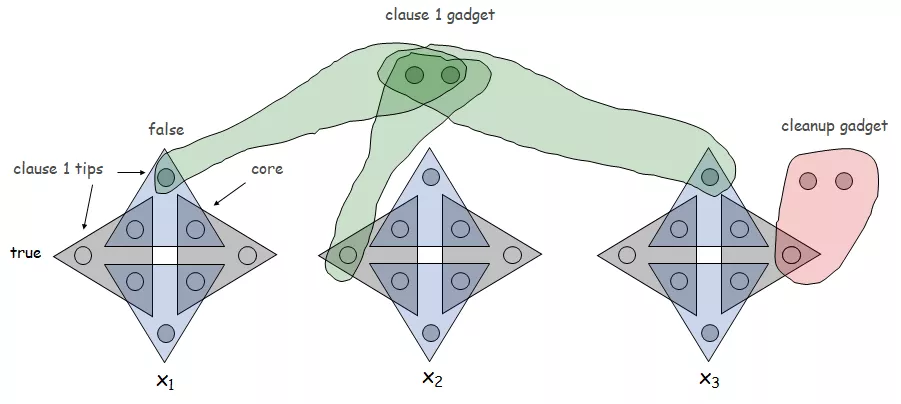Figure 11 Reduction from 3-SAT to three-dimensional matching, part two
At this time, we make:
X={a_{i,j}(j is an even number)} \vee{p_j} \vee {q_j}.
Y={a_{i,j}(j is an odd number)} \vee{p_j’} \vee {q_j’}.
Z={b_{i,j}}.
T={all constructed triples}.
It can be seen that the sizes of the three sets of X, Y, and Z are equal.
[For each 3-SAT instance]
Assuming that there is a true value assignment, if x_i=1, all odd triples of variable parts corresponding to x_i are selected. If c_j contains x_i, then c_j is 1, then (p_j,p_j’,b_{t,2j}) is selected, because b_{t,2j} is an even tip, so there is no Is covered. If x_i=0, select all even triples of variable parts corresponding to x_i. If c_j contains \overline x_i, then c_j is 1, then (p_j,p_j’,b_{t,2j-1}) is selected, because b_{t,2j-1} It is an odd tip, so it is not covered. The remaining unselected tip tuples are covered by cleanup parts.
So in the end all elements are only covered once.
So a perfect three-dimensional match is:
T={Triple selected in variable}\vee{Triple selected in clause}\vee{Triple selected in cleanup}
[For a 3D matching example]
If there is a perfect three-dimensional match, one of the 3 tuples in each clause part must be selected, and c_j is 1. If there is an even tip in the selected clause triplet, the value of the item is 1, otherwise, the value of the item is 0. That is, there is a satisfiable true value assignment. At this time, the proof of 3-SAT to three-dimensional matching has been completed. Therefore, the three-dimensional matching problem is an NPC problem.

### 6.3 Graph coloring problem

【Graph coloring problem】
In the graph coloring problem, try to assign a color to each node in the graph G, so that if (u,v) is an edge, the colors of the two nodes of the edge are different. The goal is to do this with a few colors. The number of colors used is k. The problem of graph coloring can be formulated as: arbitrarily give graph G and bound k, and ask whether G has k-coloring?

### 6.4 The three-coloring problem is NP-complete

There is a graph G that is two-colorable if and only if it is a bipartite graph (not aligned here for proof). For the three colors, the situation is more complicated. The three-coloring problem is actually an NP complete problem. First, it is easy to prove that it is an NP problem. Here, by proving 3-SAT\leq_p three-coloring, to prove that the three-coloring problem is NPC.
【3-SAT\leq_pthree coloring】
First, for each variable, add nodes v_i and \overline v_i. Add nodes T (true node), F (false node), and B (base point). Use edges to connect each pair of nodes v_i and \overline v_i. Then connect these nodes and base points. Also connect the true node, false node, and base point. As shown in Figure 12.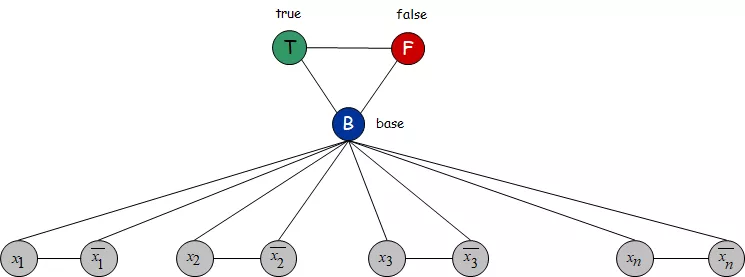Figure 12 The beginning of the reduction of the three-coloring problem
Therefore, in any three-coloring of G, the nodes v_i and \overline v_i must have different colors, and must be different from the color of the base point.
In any three colors of G, the true node, the false node and the base point must get all three colors in a certain order. According to the principle of what color can be obtained from which node, the three nodes can get the true color, false color and base color respectively. Therefore, only one of v_i and \overline v_i gets the true color, and the other gets the false color.
Consider the clause x_1 \vee \overline x_2 \vee x_3, that is, at least one of the three nodes x_1, \overline x_2, x_3 is true color. Now insert a small subgraph so that any three colors that can be extended to the small subgraph must have at least one true color for x_1, \overline x_2, x_3.
Such a sub-picture is shown in Figure 13.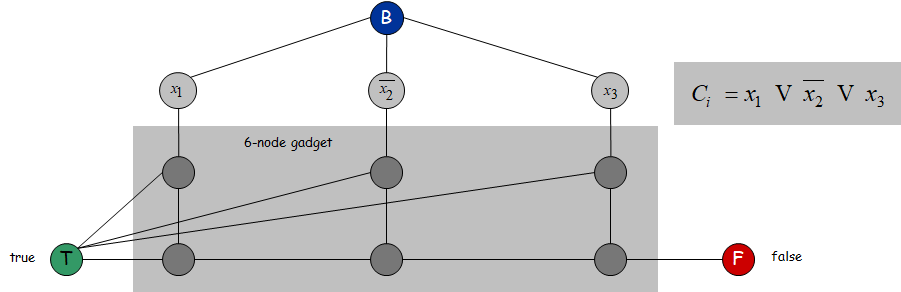Then, at least one true color must be given to x_1, \overline x_2, x_3 to make the sub-picture achieve three colors (otherwise color conflicts will occur). Figure 14 shows the three-coloring example of the subgraph.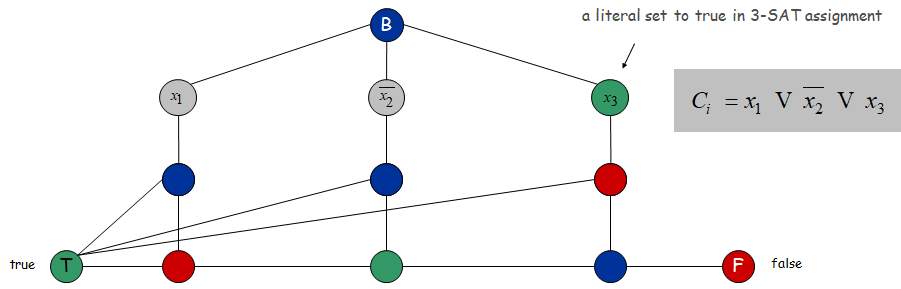Figure 14 The three-color scheme of the subgraph
It can be demonstrated that the 3-SAT instance can be satisfied if and only if the subgraph has a three-color scheme. That is, 3-SAT\leq_p has three colors, so the problem of three colors is NPC.

## 7 Numerical problems

### 7.1 Subsets and questions

【Subsets and Questions】
Given the natural numbers w_1,w_2,…,w_n and the target value W, ask {w_1,w_2,…,w_n} Is a subset of {w_1,w_2,…,w_n} exactly equal to W?
Ex: {1, 4, 16, 64, 256, 1040, 1041, 1093, 1284, 1344 }, W = 3754.
Yes. 1 + 16 + 64 + 256 + 1040 + 1093 + 1284 = 3754.

### 7.2 Subsets and problems are NP-complete

First prove that the subset sum problem is NP. Given a natural number and target W, directly find the sum of the elements in the subset to determine whether it is equal to W. Therefore, the correct answer can be verified in polynomial time.
It can be proved that the three-dimensional matching \leq_p subset sum is an NPC problem.
[3D matching \leq_p subset sum]
For each element t_a=(x_i,y_j,z_k) in the three-dimensional matching T, create a 3n (n is the number of natural numbers) d-base string (d=m+1), 3n bits correspond 3n elements of X, Y, Z. Set the ith bit, n+j bit, 2n+k bit to 1, and other bits to 0. Expressed in decimal, then w_n=d_{i-1}+d_{n+j-1}+d_{2n+k-1}. Therefore W={w_1,w_2,…,w_n}. The d system is used to prevent carry.
Therefore, we can set k=A=\sum_{i=0}^{3n-1}d_{i}.
The reduction is complete. It can be proved that there is a perfect three-dimensional match if and only if the subset and the subset in the problem exist.
Proof: Suppose there is a perfect three-dimensional match T={t_1′,t_2′,…,t_n’}, and the size of T is n. Each element t_i in T corresponds to an element w_{t_i} in W’. Because all the elements only appear once in the three-dimensional matching, so all the elements in W are added together to get a string with 3n bits and all ones, then \sum_{w \in W’}w=A.
At the same time, suppose there is a subset W’ \subseteq W such that \sum_{w \in W’}w=A. For any element w \in W’, after converting to d base, there are 3 bits as 1, corresponding to a certain element of T. These elements form a set T. T contains each element of X, Y, and Z exactly once, so T is a perfect match.

## 8 Partial classification of difficult questions

### 8.1 Packaging issues

Packaging problems mainly include independent set problems and collective packaging problems.
(1) Independent set problem: Given a graph G and a number k, does G contain an independent set with a size of at least k?
(2) Set packing problem: Given a set of n elements U, a subset of U S_1,S_2,…,S_m and the number k, ask that these subsets contain at least k sets are not intersecting?

### 8.2 Coverage issues

Covering problems mainly include vertex cover and set cover.
(1) Vertex cover: Given a graph G and number k, does G contain a vertex cover of size k?
(2) Set coverage: Given a set of n elements U, subsets of U S_1,…,S_m and the number k, there is a set of subsets in these subsets, which are equal to the entire U and contain at most k Subsets?

### 8.3 Division problem

The division problem is mainly three-dimensional matching problem and graph coloring problem.
(1) Three-dimensional matching problem: Given three disjoint sets X, Y, Z, the size of the three sets is n. Given a set of triples, the size of the set T is m. Question: Is there a subset T’of size n in T that happens to contain each element of X, Y, and Z once?
(2) Graph coloring problem: Give graph G and bound k arbitrarily, and ask whether G has k-coloring?

### 8.4 Sorting problem

The sorting problems are mainly the itinerant salesperson problem, the Hamilton circle, and the Hamilton path problem.
(1) Touring salesperson question: Given the set of distances between n cities and the limit D, is there a route that does not exceed D in length?
(2) Hamiltonian cycle: Given a directed graph G, ask whether G has a Hamiltonian cycle?
(3) Hamiltonian path: Given a directed graph G, ask whether G has a Hamiltonian path?

### 8.5 Numerical problems

Numerical problems are mainly subsets and problems.
(1) Subset sum: Given natural numbers w_1,w_2,…,w_n and target value W, ask {w_1,w_2,…,w_n} whether there is a subset that adds up to exactly W ?

### 8.6 Constraint Satisfaction Problem

Constraint satisfaction problems are mainly circuit satisfiability problems, SAT and 3-SAT.
(1) Circuit satisfiability: Given a circuit, it is necessary to determine whether there is an assignment to the input so that the output value is 1. If there is such an assignment, the circuit is said to be satisfactory. This assignment is also called a satisfying assignment.
(2) SAT: Given a set of clauses C_1,C_2,…,C_n on the set of variables X={{x_1,x_2,…,x_n}}, ask if there is Is it true value assignment?
(3) 3-SAT: Given a set of variables X={{x_1,x_2,…,x_n}}, a set of clauses C_1,C_2,…,C_n, each The length of the clause is 3. Is there a satisfactory truth assignment?

0